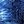Transistor ModulatorWeird Circuits wrote 03/30/2020 at 04:40 • 2 min read • Like

This paper describes a modulator made from four transistors.

This circuit is basically a voltage controlled amplifier (VCA) connected to sine wave generator that modulates the input frequency. Nowadays there are voltage controlled amplifier ICs (integrated circuits) and complete circuits sold online.

However, this circuit works at voltages as low as 1.5 V.

There is another cheaper and simpler circuit on this website. However, that circuit that only allows basic nonlinear modulation:

Step 1: Design the Circuit

I have drawn the circuit in the old PSpice software:

The 50 Hz modulator frequency is 20 times smaller than the 1 kHz circuit input frequency. Thus the signals would not overlap in the frequency domain.

I used a current mirror source to ensure a constant and temperature independent current supply to Q1a and Q1b transistors because it is the current that controls the differential amplifier AC voltage gain.

The differential voltage gain is equal to:

Gain = Vc / Vi = Beta*Rc2 / (2 * Beta * re + Ri1 + Ri2) = Rc2 / (2 * Vbe / Ie + (Ri1 + Ri2)/Beta)

(We ignore the biasing resistors Rb1 and Rb2 because they take very little current from input)

= Rc2 / (2 * Vbe / ((Vs - Vbe2) / Rm / 2) + (Ri1 + Ri2) / Beta)

= Rc2 / (4 * Vbe / ((Vs - Vbe2) / Rm) + (Ri1 + Ri2) / Beta)

= 1000 ohms / (4 * 26 mV / ((1.5 V - 0.7 V)/1000 ohms) + 1000 ohms / 100 + 1000 ohms / 100)

= 1000 ohms / 150

= 6.66666666667

For 30 mV input the output should be

Vc = Vin * Gain = 30 mV * 6.66666666667 = 200 mV or 0.2 V

Step 2: Simulations

The graph below is showing that the modulator is working.

The graph above shows the voltage controller amplifier gain output is swinging from 250 mV to 100 mV amplitude. Thus the average output amplitude is 175 mV that almost similar to the 200 mV that was predicted in the calculations. Discrepancy was caused by differences in transistor models parameter values used in the PSpice software and the model parameters values that I used in the calculations. Those parameters include the Beta value and the 26 mV input AC Vbe (AC base emitter) voltage.

Discussions